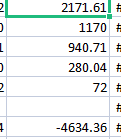cancel
Showing results for
Did you mean:Post Prodigy

## Wrong sum in total

Hello,

I am getting wrong sum in table visual:Values and sumIn excel I am getting 0 in power BI 1839. So how to resolve this issue? I also used this measure:

``sum_fix = IF(HASONEFILTER('N08-Klientai'[N08_PAV]),[day_range6],SUMX(VALUES('N08-Klientai'[N08_PAV]),[day_range6]))``

And still total sum is wrong.

2 REPLIES 2Community Support

Hi @Analitika ,

``````total_Measure =
SUMX(
SUMMARIZE(
'Table',
'Table'[RowName],
'Table'[ColumnName],//If there are no rows or columns, please delete
),
[total]
)
``````

Best Regards,

Neeko Tang

If this post  helps, then please consider Accept it as the solution  to help the other members find it more quickly.Responsive Resident

Watch this to fix incorrect total in measure.

How to fix Incorrect Measure Total in PowerBI | MiTutorials - YouTube